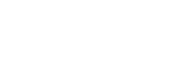Supervised Contrastive Learning这篇论文在有监督学习、交叉熵损失与有监督对比损失之间进行了大量的讨论，以更好地实现图像表示和分类任务。让我们深入了解一下这篇论文的内容。```Code
self.encoder = resnet50()
def forward(self, x):
feat = self.encoder(x)
#需要对128向量进行标准化
return feat``````#尝试理解下面的代码
import numpy as np
v = np.random.randn(128)
v = v/np.linalg.norm(v)
print(np.dot(v,v))
print(np.linalg.norm(v))```8=4×2的原因是我们对每个图像总是有一个对比度，因此需要相应地编写一个数据加载程序。

```#batch大小
bs = 4``````temperature = 0.07
anchor_feature = contrast_feature
anchor_dot_contrast = torch.div(
torch.matmul(anchor_feature, contrast_feature.T),
temperature)```anchor_feature=3×128和contrast_feature=128×3，结果为3×3，如下所示```#bs 1 和 dim 2 意味着 2*1x2
features = torch.randn(2, 2)
temperature = 0.07
contrast_feature  = features
anchor_feature = contrast_feature
anchor_dot_contrast = torch.div(
torch.matmul(anchor_feature, contrast_feature.T),
temperature)
print('anchor_dot_contrast=\n{}'.format(anchor_dot_contrast))
logits_max, _ = torch.max(anchor_dot_contrast, dim=1, keepdim=True)
print('logits_max = {}'.format(logits_max))
logits = anchor_dot_contrast - logits_max.detach()
print(' logits = {}'.format(logits))
#输出看看对角线发生了什幺
anchor_dot_contrast=
tensor([[128.8697, -12.0467],
[-12.0467,  50.5816]])
logits_max = tensor([[128.8697],
[ 50.5816]])
logits = tensor([[   0.0000, -140.9164],
[ -62.6283,    0.0000]])```

```bs = 4
print('batch size', bs)
temperature = 0.07
labels = torch.randint(4, (1,4))
print('labels', labels)
#对它进行硬编码，以使其更容易理解
contrast_count = 2
anchor_count = contrast_count
#屏蔽self-contrast的情况
1,
torch.arange(bs * anchor_count).view(-1, 1),
0
)

```batch size 4
labels tensor([[3, 0, 2, 3]])
#以上的意思是在这批4个品种的葡萄中，我们有3,0,2,3个标签。以防你们忘了我们在这里只做了一次对比所以我们会有3_c 0_c 2_c 3_c作为输入批处理中的对比。
tensor([[0., 1., 1., 1., 1., 1., 1., 1.],
[1., 0., 1., 1., 1., 1., 1., 1.],
[1., 1., 0., 1., 1., 1., 1., 1.],
[1., 1., 1., 0., 1., 1., 1., 1.],
[1., 1., 1., 1., 0., 1., 1., 1.],
[1., 1., 1., 1., 1., 0., 1., 1.],
[1., 1., 1., 1., 1., 1., 0., 1.],
[1., 1., 1., 1., 1., 1., 1., 0.]])

# 所以我们的标签就是标签张量([[3,0,2,3]])
# 我重新命名它们是为了更好地理解张量([[3_1,0_1,2_1,3_2]])
tensor([[0., 0., 0., 1., 1., 0., 0., 1.],
[0., 0., 0., 0., 0., 1., 0., 0.],
[0., 0., 0., 0., 0., 0., 1., 0.],
[1., 0., 0., 0., 1., 0., 0., 1.],
[1., 0., 0., 1., 0., 0., 0., 1.],
[0., 1., 0., 0., 0., 0., 0., 0.],
[0., 0., 1., 0., 0., 0., 0., 0.],
[1., 0., 0., 1., 1., 0., 0., 0.]])````logits = anchor_dot_contrast — logits_max.detach()````#上述等式的第二部分等于torch.log(exp_logits.sum(1, keepdim=True))
log_prob = logits - torch.log(exp_logits.sum(1, keepdim=True))
# 计算对数似然的均值
# 损失
loss = - mean_log_prob_pos
loss = loss.view(anchor_count, 4).mean()
print('19. loss {}'.format(loss))```

#### 参考引用

 : Supervised Contrastive Learning
 : Florian Schroff, Dmitry Kalenichenko, and James Philbin. Facenet: A unified embedding for face recognition and clustering. In Proceedings of the IEEE conference on computer vision and pattern recognition, pages 815–823, 2015.
 : A Simple Framework for Contrastive Learning of Visual Representations, Ting Chen, Simon Kornblith Mohammad Norouzi, Geoffrey Hinton